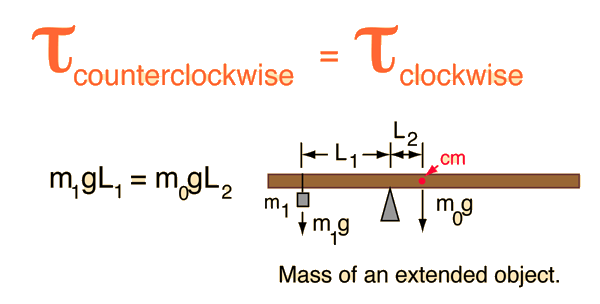# Determining the Mass of an Extended Oject

The mass of an extended object can be found by using the conditions for equilibrium of torques. If the object is first balanced to find its center of mass, then the entire weight of the object can be considered to act at that center of mass. If the object is then shifted a measured distance away from the center of mass and again balanced by hanging a known mass on the other side of the pivot point, the unknown mass of the object can be determined by balancing the torques.But since g appears on both sides of the equation, it can be factored out and just the masses and lengths used for the calculation.

m1L1 = m0L2
mass1 x lever1 = mass of rod x lever2

kg x m = kg x m
You may enter data for any of the quantities above. When finished entering data, click on the active text for the quantity you wish to calculate. The data values will not be forced to be consistent until you click on the desired quantity.
Index

Torque equilibrium examples

 HyperPhysics***** Mechanics R Nave
Go Back